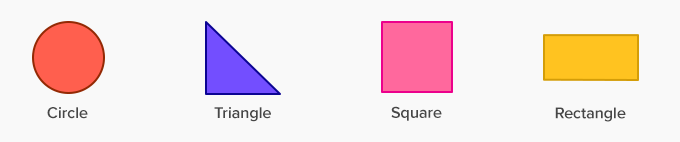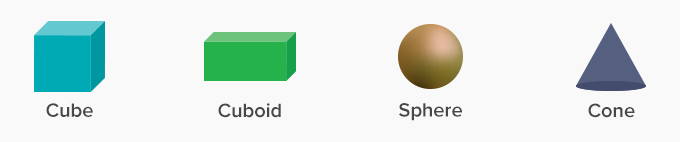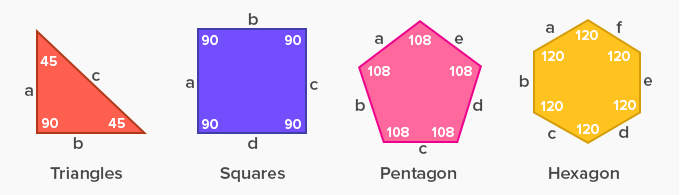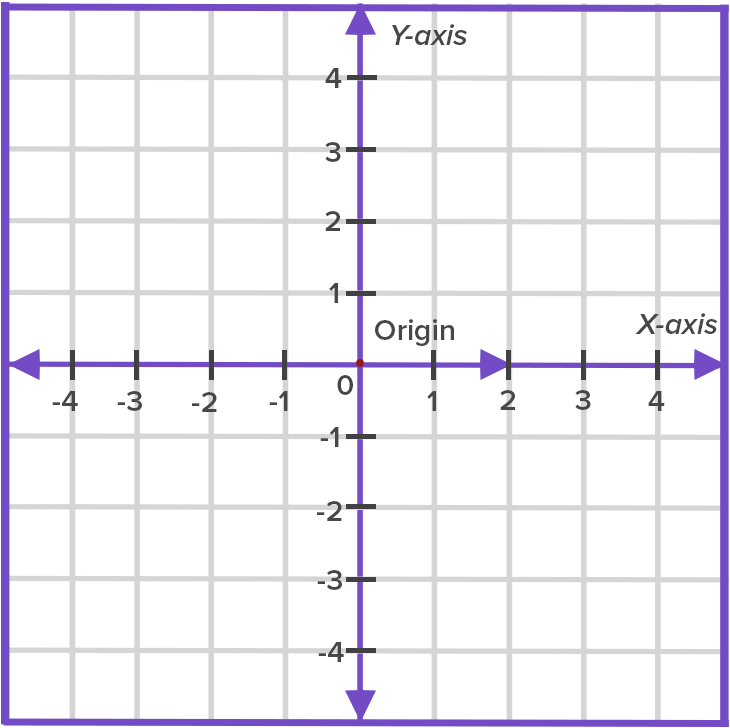Geometry - Definition with Examples

The Complete K-5 Math Learning Program Built for Your Child

• 30 Million Kids

Loved by kids and parent worldwide

• 50,000 Schools

Trusted by teachers across schools

• Comprehensive Curriculum

Aligned to Common Core

What is Geometry?

Geometry is a branch of mathematics that studies the sizes, shapes, positions angles and dimensions of things.

Flat shapes like squares, circles, and triangles are a part of flat geometry and are called 2D shapes. These shapes have only 2 dimensions, the length and the width.

Examples of 2D shapes in flat geometrySolid objects are also known as 3D objects having the third dimension of height or depth.

Examples of 3D shapes in solid geometryAngle:

The vertex of a shape where two edges meet form an angle. Different shapes in geometry have different angle measures.

For example:

• A triangle is a 3 sided shape, and the measure of its 3 interior angles is 180˚

• A square, rectangle or quadrilateral are 4 sided shapes, and the measure of their interior angles is 360˚

• Other polygons like the pentagon, hexagon, heptagon, octagon have 5, 6, 7, 8 sides respectively and varying angles.

Examples of different Polygons with their angles and sidesWe learn various aspects of shapes, like the measurement of angles, length of sides, area, volume, etc in geometry.

Similarity and congruence are two important aspects of geometry.

Similarity: Similarity is when two shapes are the same but their sizes may vary.Congruence: Congruence is when two shapes are exactly the same in shape and size.

Coordinate Plane:

• A coordinate plane is a 2D surface formed by using two number lines that intersect each other at the right angle.

• The horizontal number line is the x-axis, and the vertical number line is the y-axis.

• The intersection of the two axes is the (0,0) coordinate.

• Using the coordinate plane, we plot points, lines, etc. By joining various points on the coordinate plane, we can create shapes.We use Formula and Theorems to solve the geometry problems.

A formula is a mathematical equation to solve a geometry problem while a theorem is a statement that is proved using previously known facts.

For example, the "Pythagoras Theorem" proved that a2 + b2 = c2 for a right-angled triangle, where a and b are the sides of the right-angled triangle, and c is the hypotenuse.However, a2 + b2 = c2 is the formula for finding the hypotenuse of a right-angled triangle.

 Fun Facts The word geometry is made from the Greek words “Geo” meaning “earth” and “metry” meaning “measurement”.

Won Numerous Awards & Honors